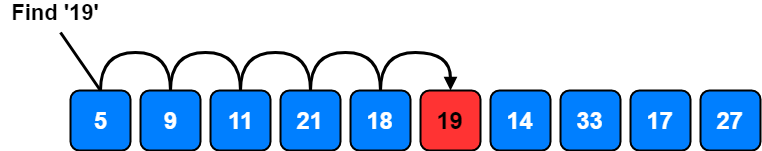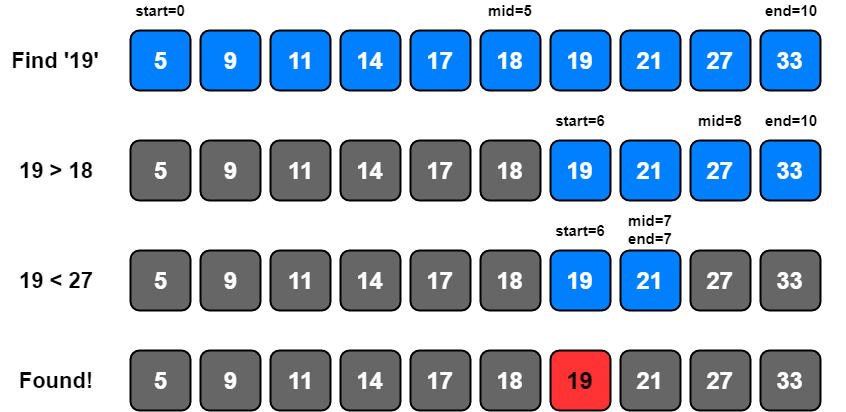# Search Algorithms

Searching for items and sorting through items are tasks that we do everyday. If a deck of cards has less than 52 cards, how do you determine which card is missing? How do you find your keys when you have misplaced them?

Searching and sorting are also very common and well-studied tasks in Computer Science. Computers are often required to find information in large collections of data. Think about a search engine which searchs a specific query word in billions of web pages. It sure is harder than finding a missing card in a card deck! They need to develop quick and efficient ways of doing this.

There are lots of different search algorithms but we can divide them in to two basic types: algorithms that don’t make any assumptions about the order of the list, and algorithms that assume the list is already in order.

Linear search (a.k.a Sequential Search) is the simplest search algorithm. In this algorithm, we are looking at each item in the list one-by-one until we find an item that matches the search term or we have reached the end of the list. If we found a matching item, we are returning its index value.### Linear Search Implementation

``````def linear_search(arr, key):
for i in range(len(arr)):
if arr[i] == key:
return i
return -1
``````

A for loop iterates through the list, when an item matching the search key is found, the corresponding index is returned. If no such search key is found in the list, -1 is returned.

### Performance

To be able to measure the performance of an algorithm, we need to look at three cases:

• Best case: occurs when search term is found in the first slot of the array. Number of total comparisons in this case is 1
• Worst case: occurs when search term is found in the last slot of the array or search term is not in the array. Number of total comparisons in this case is equal to the size of the array. If our array has N items then it takes N comparisons in the worst case.
• Average case: On average, the search term will be found somewhere in the middle of the array. The number of total comparisons in this case is approximately N/2

In short we can say that, performance of Linear Search is O(1) for the best case and O(N) for the average case and worst case.

Linear search works well if our list is not in order but it can be slow if there are so many number of items in our list. What if we are sure that our list is already in order?

Binary search exploits the ordering of the list. The idea behind is, each time we made a comparison, we should eliminate the half of the list until we either find the search term or determine that the term is not in the list. We do this by looking at the middle item in the list, and determining if our search term is higher or lower than this middle item.### Binary Search Implementation without Recursion

``````def binary_search(arr, key):
start = 0
end = len(arr)
while start < end:
mid = (start + end)//2
if arr[mid] > key:
end = mid
elif arr[mid] < key:
start = mid + 1
else:
return mid
return -1
``````

start variable is set to 0 and end variable is set to the length of the list. A while loop iterates as long as start variable is less than the end variable. mid variable is calculated as the floor of the average of start and end.

If the element at index mid is less than search key, it means that our search key is in the 2nd part of the list so, start is set to mid + 1 and if it is more than key, it means that our search key is in the 1st part of the list so, end is set to mid.

Otherwise, mid is returned as the index of the found element. If no such item is found, -1 is returned.

### Binary Search Implementation with Recursion

``````def recursive_binary_search(arr, start, end, key):
if not start < end:
return -1

mid = (start + end) // 2
if arr[mid] < key:
return recursive_binary_search(arr, mid + 1, end, key)
elif arr[mid] > key:
return recursive_binary_search(arr, start, mid, key)
else:
return mid
``````

Our base case is testing whether start is less than end. If not, -1 is returned.

mid variable is calculated as the floor of the average of start and end.

If the element at index mid is less than search key, `recursive_binary_search()` is called again with `start = mid + 1` and if it is more than search key, it is called with `end = mid`.

Otherwise, mid is returned as the index of the found element.

### Performance

• Best case: occurs when search term found in the first try. In this case, the search term would be the middle item in the list
• Worst case: The worst case is, when search term is not in the list, or when search term is one item away from the middle item or when search term is first or last item in the list.
• Average case: occurs when search term is anywhere else in the list

So, we can say that, performance of Binary search is O(1) for the best case and O(logN) for the average case and worst case which is much better than Linear Search!

## Others

Linear Search and Binary Search are very basic and useful search algorithms that you will need to know right away. But there are other search algorithms like; Depth-First Search (DFS)2, Breadth-First Search (BFS)3, Jump Search4, Ternary Search5, Interpolation Search6, Exponential Search7, Fibonacci Search8, Rabin-Karp Pattern Search9, Knuth-Morris-Pratt Pattern Search10, etc.

I will explain some of these algorithms in separate posts.

1. Carleton College, CS 117: Introduction to Computer Science
2. Wikipedia, Depth-First Search (DFS)